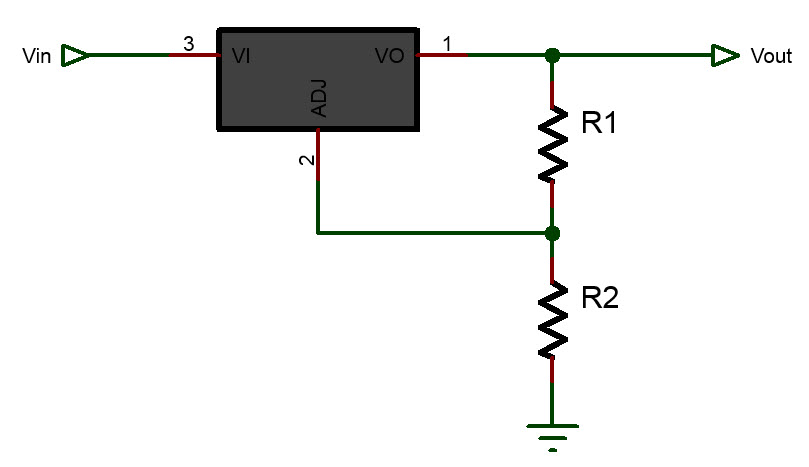# LM317 Voltage Regulator Online Calculator

Calculate resistor R2:
V

Theoretical Results:Ajustable LM317 Voltage Regulator Calculator

The Adjustable LM317 Voltage Regulator Calculator is a useful tool for electronics enthusiasts and professionals who need to design or troubleshoot circuits that use the LM317 voltage regulator. This calculator allows users to quickly and easily calculate the values of the resistors they need to use in their LM317 voltage regulator circuits to achieve a desired output voltage. To use the calculator, the user simply needs to enter the desired output voltage and the value of resistor R1. The calculator then calculates the value of the second resistor (R2) that is needed to achieve the desired output voltage, along with the power dissipation of the LM317 and the power rating of R1 and R2. The calculator also displays the closest standard resistor values that can be used. This calculator can be particularly useful for engineers, hobbyists, and students who are working on electronic projects that require a voltage regulator. For example, it can be used to design power supplies, battery chargers, or LED drivers. It can also be used to troubleshoot existing circuits that are not functioning properly, by allowing the user to quickly calculate the values of the resistors that are needed to adjust the output voltage. By making this calculator available online, users can easily access it from anywhere with an internet connection, and it can be used on various devices such as desktop computers, laptops, tablets, and smartphones. Additionally, since the calculator is web-based, users don't need to download any software or plugins to use it. Overall, the Adjustable LM317 Voltage Regulator Calculator is a useful and convenient tool for anyone working with LM317 voltage regulators. It simplifies the process of calculating the values of resistors needed for a particular output voltage, saving users time and effort in their projects.

LM317 Voltage Regulator formula or equation:

$V_{out} = 1.25(1+ \frac{R_1}{R_2})$,
$R_2= \frac{V_{out}R_1}{1.25} - R_1$